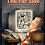## Monday, September 26, 2011

### 5 different ways to add all the numbers in a file

In this article, we will see the different ways in which can add or sum up all the contents in a file.

Let us take a sample file with the following contents:
```\$ cat file
3
4
5
```

1. The simplest of all is using the awk method.
```\$ awk '{x+=\$0}END{print x}' file
12
```
When every line is processed, the number is being added to the variable x. And once the end of the file(END) is reached, we simply print the variable x.

2. The second one is using the paste command along with the bc:
```\$ paste -s -d"+" file | bc
12
```
The "-s" option in paste joins all the lines of a file. The "-d" options lets us to choose the delimiter with the lines are to be joined. At the end of the paste command, we end up with: "3+4+5", which when passed to the bc command gives the result.

3. The next solution is using the perl command which has the same logic like the awk one:
```\$ perl -lne '\$x+=\$_; END{print \$x;}' file
12
```
The \$_ indicates the current line in perl, as in \$0 for awk. The variable \$x is printed once the end of the file is reached.

4. sed solution is a bit tricky.
```\$ sed -e :a -e 'N;s/\n/+/;ta' file | bc
12
```
This sed command joins all the lines(N) and separates them with the '+'. At the end of sed, we end up with "3+4+5" which when passed to bc gives us the requisite result.

5. The last one is using the echo command in place of bc to do the addition:
```\$ echo \$((`sed '\$!s/\$/+/' file | tr '\n' ' '`))
12
```
The sed command puts a '+' at the end of each line, except for the last line(\$!). And the 'tr' command removes the new line. echo does the addition.

1.2.